### James S. Clark, Michael Wolosin, Michael Dietze, Inés Ibáñez, Shannon LaDeau, Miranda Welsh, and Brian Kloeppel. 2007. Tree growth inference and prediction from diameter censuses and ring widths. Ecological Applications 17:1942–1953.

Appendix A. Tree growth inference and prediction.

Gibbs sampling is a Markov chain Monte Carlo (MCMC) technique that involves alternate sampling from each of the full conditional distributions of all unknowns in the model, including parameters and latent variables (Gelfand and Smith 1990, Clark 2005). Here we provide conditional distributions, together with algorithms used to sample from them. In this appendix, p(g) designates the value of parameter p in the gth step of the Gibbs sampler, and p* is a proposed value.

The conditional distribution of growth increments for individual i in stand j is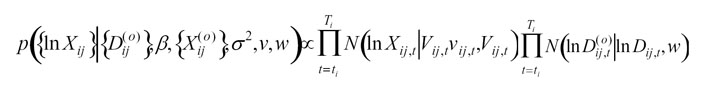where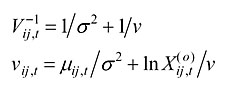for years in which growth increment observations are available, and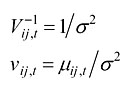for years in which increment observations are unavailable. Likewise, the density for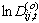is included only for years in with diameter measurements are available. The solutions for these conditional distributions are provided in Clark (2007). A Metropolis sample from this distribution begins with a proposal for the first diameter value from a lognormal density that is truncated at two cm in width centered on the first observation. Then all diameter increments are proposed from a lognormal (to insure that they are positive) for the years in which individuals were alive and included in the study. Proposals are accepted on a tree-by-tree basis. In other words, blocking is by tree, but there are no loops over trees or years.

Parameters involved in Eqs. 1 and 2 are all sampled directly from conditional Gaussian densities. For the overall mean growth rate, the conditional posterior, integrated over random effects, is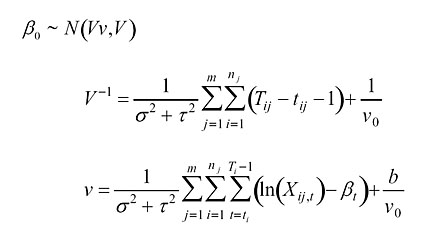where nj is the number of trees in stand j. Random individual effects are sampled from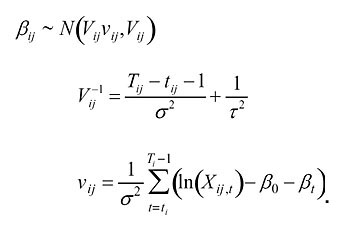Year effects are sampled from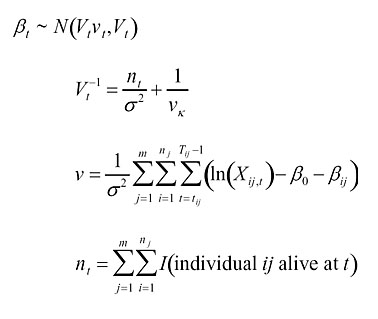where I(A) is the indicator variable equal to one, when event A is true, and zero, otherwise. We impose a sum-to-zero constraint on the year effects by subtracting the mean at each Gibbs step.

Variance parameters are all directly sampled from inverse gamma distributions. These are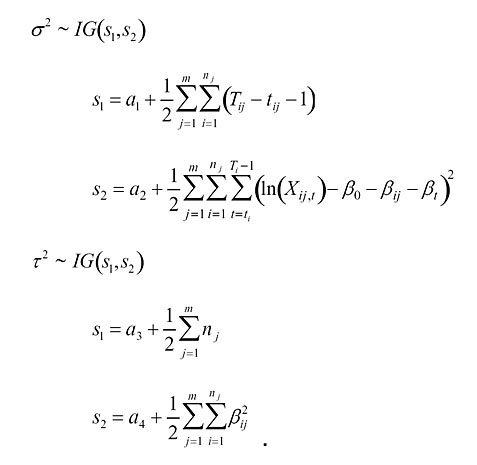Observation errors on growth are sampled from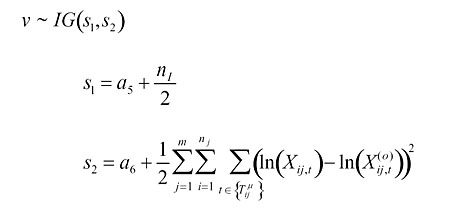where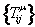is the set of growth observations on tree ij. Observation errors on diameter are sampled from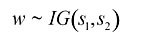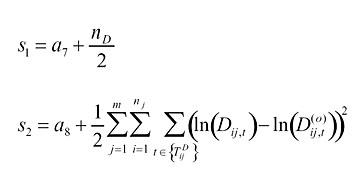where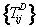is the set of diameter observations on tree ij.

Because increment cores can shrink with moisture loss, a bias parameter can be included in the data model. A multiplicative bias on diameter increment (additive on log diameter increment is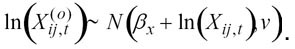Then the conditional density has parameters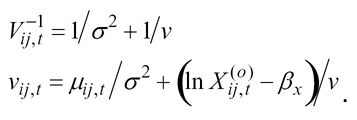The conditional density for the bias parameters is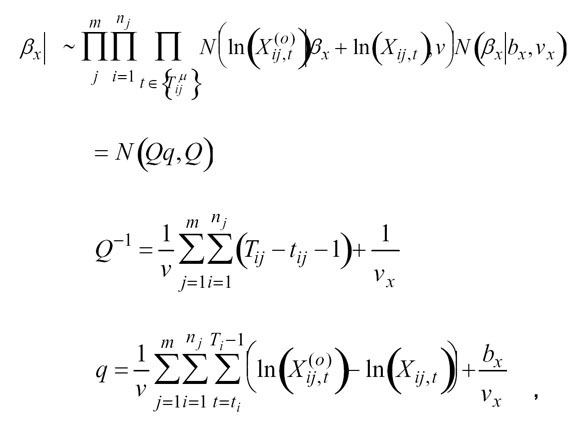and the error conditional is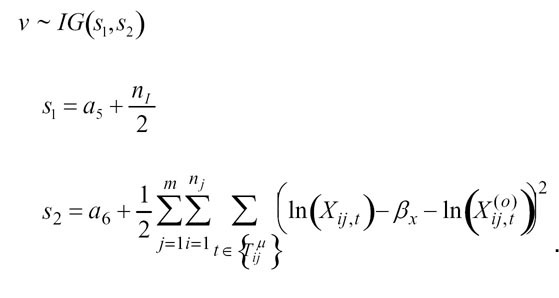We do not prove convergence, but extensive simulation indicates that the algorithm does converge to appropriate estimates. The latent diameter states were initialized by interpolation, subject to the constraint that there can be no decreasing diameters. The latent growth rates are initialized as the differenced initial diameter series.

The Gibbs sampler was run 500,000 iterations to insure convergence and then run an additional 500,000 iterations to obtain posterior estimates. Because of the large number of estimates (11,444 tree-years), we did not retain all 500,000 times 19,840 estimates each for diameter and growth. Rather, we retained the sums and the sums of the squared diameter and log growth estimates from every 20th Gibbs step. Because both variables were approximately Gaussian, 95% credible intervals were calculated as + 1.96 standard deviations, which came, in turn, from first and second moments. These are the intervals shown in Figs. 4 and 5. For all other parameters credible intervals are obtained as percentiles of the Gibbs output.

Predictive loss Dm is the sum of a goodness of fit term and a penalty term,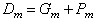This can be done for both data sets. For diameter measurements, goodness of fit is taken to be the error sum of squares about the predictive mean diameter,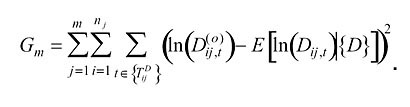The model is penalized for imprecise predictions, given by the predictive variance,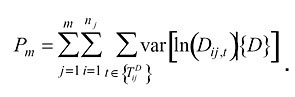The quantities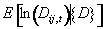and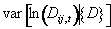are the predictive means and variances that are obtained from repeated simulations of Dij,t beginning with a draw from the posterior for the first diameter in year tij and ‘growing’ the tree, each time based on different parameter vector taken from the Gibbs output (Clark 2007).

LITERATURE CITED

Clark, J. S. 2005. Why environmental scientists are becoming Bayesians. Ecology Letters 8:2–14.

Clark, J. S. 2007. Models for Ecological Data. Princeton University Press. Princeton, New Jersey, USA.

Gelfand, A. E., and A. F. M. Smith. 1990. Sampling-based approaches to calculating marginal densities. Journal of the American Statistical Association 85:398–409.

[Back to A017-077]